Next: Elimination of ambiguity. Up: Context-free grammars Previous: Context-free grammars

## Parse trees

PARSE TREES. Let G = (VT, VN, S, P) be a context-free grammar. A parse tree for G is a tree constructed as follows:

• its root is labelled by the start-symbol S of G,
• each internal node is labeled by a non-terminal,
• the leaves are labeled by terminals or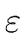, the empty-word symbol,
• if an internal node is labeled by a non-terminal A and its children from left to right are labeled by grammar symbols X1,..., Xn then

 A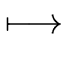X1 ... Xn (16)

is a production of G. Moreover if n = 1 we can have X1 =.
Let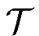be a parse tree. The string obtained by concatenating the labels of the leaves offrom left to right id called the YIELD of the parse tree.

Let w be a word of the language generated by G. Clearly there exists at least one parse tree for G whose yield is w. Such a tree is called a parse tree for w w.r.t. G.

LEFTMOST/RIGHTMOST DERIVATIONS. Let G = (VT, VN, S, P) be a context-free grammar. The derivation (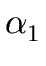,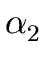,...,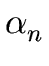) is LEFTMOST if for each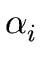(with i = 1 ... n - 1) the leftmost non-terminal is rewritten. Analogously we define rightmost derivations.

Remark 3   A parse tree does not specify the order in which productions are used to rewrite non-terminal symbols by strings of grammar symbols: a given parse tree usually represents several different derivations.

However, to any parse tree we can associate a unique leftmost (or a unique rightmost) derivation. Top-down parsing attempts to find a leftmost derivation for the input. In bottom-up parsing, we construct a rightmost derivation (in reverse order).Next: Elimination of ambiguity. Up: Context-free grammars Previous: Context-free grammars
Marc Moreno Maza
2004-12-02